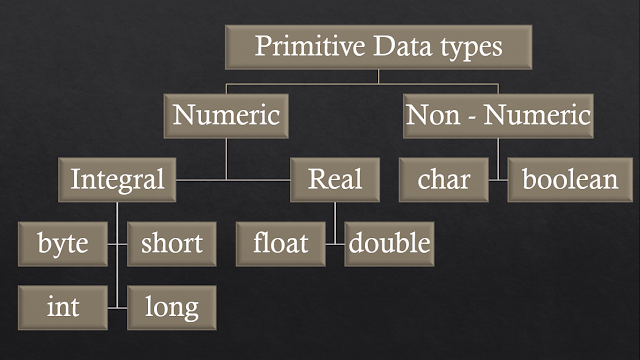## What is Data type

1. In Java every variable have some type.
2. For example in the code snippet below x should be int and b should be boolean type variable.
x = 10; b = true;
1. Similarly in Java every expression also have some type.
2. For example in the following code snippet if a + b + c would result int type result because a, b and c are int type variables.
int a, b, c, d;
a = 1; b = 1; c = 1;
d  = a + b + c;

#### Strictly type checking

1. In Java, each type is strictly defined means byte have range from -128 to 127, so value out of from this range is not acceptable by byte type.
2. Each assignment is strictly checked for type compatibility.
3. Due to above two reason java is called strongly type checked language.

## Primitive Data Types8 Primitive Data types in Java

### Signed / Unsigned Data types

#### Examples

int a = 10; ()
int a = -10; ()
float x = -5.8; ()
char ch = -'a'; (✕)
boolean b = -true; (✕)
boolean b = true; ()
1. Except boolean and char other primitive data type can be positive or negative.
2. Data types which can have positive or negative values are called signed data types.
3. Data type which can have only positive values are called unsigned data types, char and boolean are unsigned data types in Java.

## Data type Explained

### 1. byte data type

1. Size: 1 byte (8 bits)
2. Min Value: -128 (-27)
3. Max Value: 127 (27 - 1)
4. Range: -128 to 127 (-27 to 27 - 1)

### Some example of byte data type

#### Example-1:

byte b = 125; ()
System.out.println(b);

#### Example-2:

byte b = 128;(✕)
System.out.println(b);

#### Example-3:

byte b = 5.8;(✕)
System.out.println(b);

#### Example-4:

byte b = "abc";(✕)
System.out.println(b);

#### Uses of byte

If you want to handle data in terms of streams (a sequence of bits) either from the file or from the network, the best supported form is byte.

### 2. Short data type

1. Size: 2 byte (16 bits)
2. Min Value: -32768 (-215)
3. Max Value: 32767 (215 - 1)
4. Range: -32768 to 32767 (-215 to 215 - 1)
short data type is very rarely used data type.

### 3. int data type

1. Size: 4 byte (32 bits)
2. Min Value: -2147483648 (-231)
3. Max Value: 2147483647 (231 - 1)
4. Range: -2147483648 to 2147483648 (-231 to 231 - 1)
int data type is mostly used data type in Java.

#### Example-1

int x = 2147483648; (✕)

In the above example 2147483648 is out the the integer range. so error number is too large.

#### Example-2

int x = 2147483648L; (✕)

In the above example, as we suffix L/l to 2147483648 so this becomes long constants.

### 4. long data type

1. Size: 8 byte (64 bits)
2. Min Value:  -263
3. Max Value: 263 - 1
4. Range: -263 to 263 - 1

### Real data type

If we want to store floating point value, then we use floating point data type that can be either float or double data type.
if you need store floating values with more precision (accuracy) then you should use double data type otherwise use float data type.

### 5. float data type

1. Single Precision : 6-7 digit accuracy
2. Size: 4 byte (32 bits)
3. Min Value:  -1.7e38 ( -1.7 *  1038 )
4. Max Value: 1.7e38 (1.7 * 1038)
5. Range: -1.7e38 to 1.7e38

### 6. double data type

1. Double Precision : 14-15 digit accuracy
2. Size: 8 byte (64 bits)
3. Min Value:  -3.4e308 ( -3.4 *  10308 )
4. Max Value: 3.4e308 (3.4 * 10308)
5. Range: -3.4e308 to 3.4e308

### 7. boolean data type

1. Size: N/A (Depend upon JVM)
2. Range: N/A [Allowed values are false / true.]

#### Example-1

boolean b = 0;
System.out.println(b);

#### Example - 2

boolean b = "true";
System.out.println(b);

#### Example - 3

boolean b = True;
System.out.println(b);

#### Example - 4

boolean b = true;
System.out.println(b); //true

#### Example - 5

int a = 0;
if(a) {
System.out.println("Hello");
} else {
System.out.println("Bye");
}

#### 8. char data type

1. Size: 2 byte (16 bits)
2. Min Value:  0
3. Max Value: 65535 (216 - 1)
4. Range: 0 to 65535 (216 - 1)

#### Important facts about char data type

1. Old languages like C / C++ supports ASCII encoding which covers only 256 characters ranges from 0 - 255 contains only English language alphabets, digits and some special characters.
2. Java support Unicode character encoding, which represents 65536 characters ranges from 0 to 65535. Unicode character set includes alphabets, digits, special symbols and characters to represents other languages like Hindi and many other language.
3. Unicode first 255 characters are same as ASCII characters, so Unicode is called super-set of ASCII.

### Data Type Summary

Data type Size Range Default Value
byte 1 byte -128 to 127 0
short 2 byte -32768 to 23767 0
int 4 byte -2147483648 to 2147483647 0
long 8 byte -231 to 231 - 1 0
float 4 byte -1.7 * 1038 to 1.7 * 1038 0.0f
double 8 byte -3.4 * 10308  to 3.4 to 10308 0.0
char 2 byte 0 to 65535 (0)\u0000
boolean N/A true / false false